# Experimental research on hydraulic characteristic of centrifugal pump in plateau

Experimental research on hydraulic characteristic of centrifugal pump in plateau

Jiang Li*Weiming Zhang

Department of Oil, Army Logistics University of PLA, Chongqing 401311, China

Corresponding Author Email:
lijiang830@163.com
Page:
585-593
|
DOI:
https://doi.org/10.18280/ijht.350315
|
Accepted:
|
Published:
30 September 2017
| Citation

OPEN ACCESS

Abstract:

The hydraulic characteristics of a centrifugal pump change when in a plateau, while the corresponding law is unclear. Therefore, experimental research needs to be undertaken on the hydraulic characteristics of a centrifugal pump in a plateau for a quantitative analysis of the changing law. In this paper, the working status of a centrifugal pump in a plateau was simulated by the method of controlling the inlet vacuum so as to acquire the pressure and the flow data under different working conditions. The corresponding conclusions were reached by using thorough data analysis, which shows that with every 500m increase in altitude, the accompanying pump pressure decreased by about 5%-10%, the flow decreased by about 2%-5% and the overall efficiency decreased by about 2%-9%. This theoretical analysis shows that the increase in pump frictional loss is the fundamental reason for the change in the hydraulic characteristics of a centrifugal pump in a plateau.

Keywords:

experimental research, plateau, centrifugal pump, hydraulic characteristics, pressure, flow, efficiency

1. Introduction

The centrifugal pump has the advantages of high delivery pressure and large flow, and so it is widely used in oil transportation, accounting for about 70%-80% of the total pumps . Good hydraulic characteristics are of great significance to ensure reliable fluid transfer . In a plateau, the atmospheric pressure decreases along with the increase of altitude, which leads to a decrease in the pump suction capacity, because of which the normal working status of the centrifugal pump will be affected . Therefore, it is necessary to make the changing laws of the centrifugal pump’s working status in a plateau clear, and to discover the changing law of hydraulic characteristics and the factors that influence it.

Undertaking experiments is the most direct and effective method, but owing to experimental space, experimental conditions and other restrictions, it is far more difficult to carry out an experiment in a plateau, so simulation methods are often used instead . Bench simulation experiments  are common simulation methods. Thomas Schäfera  proposed the method of scanning the gas retention status in a centrifugal pump with high-penetration gamma rays, and then made a quantitative analysis of the pump gas-liquid two-phase flow distribution. Bao-cheng Shi  proposed an experimental device for measuring the internal flow of solid-liquid two-phase in a centrifugal pump based on the particle image velocity method (PIV). Through the PIV results, LI Ya-lin  estimated the tracking characteristics of various tracer particles, discussed the advantages and disadvantages of different tracer particles and proposed suitable PIV tracer particles for a centrifugal pump. Computer simulation experiments  are commonly used in addition. José A. Caridad  simulated the characteristics of centrifugal pump blades in the gas-liquid two-phase flow by CFD, and discovered its characterization. According to the velocity triangles of the centrifugal pump, Pei Ji [ 11] deduced the volume of the unsteady flow intensity, and the turbulent flow field of the unsteady flow in the low specific speed centrifugal pump was simulated. Hou-lin Liu  proposed an improved κ-ω model, which is coupled with the Schnerr-Sauer cavitation model by ANSYS CFX to predict the cavitation flow in the centrifugal pump. The results of the computer simulation experiment are easily affected by factors such as model precision, boundary conditions and related factors, and hence its accuracy cannot be determined [13-14]. Therefore, a bench simulation experiment is adopted in this paper.

In this paper, according to the relationship between altitude, atmospheric pressure and vacuum degree, a vacuum pump and vacuum tank are used to simulate the atmospheric pressure in a plateau, and then experimental research into the hydraulic characteristics of a centrifugal pump in a plateau is carried out by the method of controlling the inlet vacuum pressure. During the experiment, the hydraulic characteristics such as inlet pressure, outlet pressure and the flow of the pump under different work conditions are obtained. Based on these data, this paper posits an in-depth and detailed quantitative analysis of the changing law of pump hydraulic characteristics, and analyzes the reasons for the characteristic decline of the hydraulic pump.

This paper has been divided into three sections; the first part consists of an introduction to the experiment; the second section consists of experimental data and an analysis of the pressure, flow, efficiency and reasons that make the pump hydraulic characteristics decrease; the final part is the conclusion of this work.

2. Experimental Principles and Platform

2.1 Main equipment

Table 1. Main technical parameters of centrifugal pump

 model rated speed(r/min) rated flow(m3/h) rated pressure(MPa) rated efficiency (%) 3YT50×5 2950 45 0.025 63

Table 2. Main technical parameters of vacuum pump

 model rated speed (r/min) maximum gas volume(m3/min) ultimate vacuum(MPa) power(kW) 2BV-2070 2850 1.5 0.097 2.35

(1) Pump

Centrifugal pump and vacuum pump are mainly used. The experimental object is a five-stage centrifugal pump with non self-suction capacity driven by an engine. The main technical parameters are shown in table 1. The vacuum pump is a water ring vacuum pump driven by the motor, used to vacuumize the vacuum tank, and its main technical parameters are shown in table 2.

(2) Vacuum tank

The vacuum tank is the main equipment used to control the pump suction pressure, whose volume is 4.3m3, and whose designed pressure is 1.1Mpa; it is equipped with a vacuum gauge with a measuring range of 0Mpa -0.09Mpa, and with a measurement accuracy of 0.001Mpa.

(3) Pressure gauge

An MPM489 pressure gauge of the Hong Kong microphone company was selected to measure the pressure of the pipeline; its range is -0.1 MPa-0.3MPa with an accuracy level of 0.5%.

(4) Flow meter

An AVX-100-DDL-123 intelligent vortex flow meter was used in the experiment. The measuring range of the flow meter is 0m3/h -270m3/h, and its nominal pressure is 4MPa with an accuracy of 0.01MPa.

(5) Thermometer

In the experiment, the temperature of the fluid in the pipeline was measured by a WSS1.5 thermometer. The measuring range was 0 ℃ -100 ℃, the measurement accuracy was 0.1 ℃, and the direct reading method was adopted.

2.2 Experimental principle

## 1.pngFigure 1. Relationship between altitude and vacuum degree

The vacuum degree increases with the rise of altitude, which can be used as an important indicator to measure atmospheric pressure. The corresponding relationship between the vacuum degree and the altitude is shown in Figure 1 .

There is a linear relationship between the altitude and the vacuum referring to figure 1; the vacuum pump and vacuum tank are used to simulate the atmospheric pressure at different altitudes, and the vacuum tank and engine pump unit are connected into a closed loop, so that experimental research of the flow state in the centrifugal pump is carried out by controlling the inlet vacuum degree of the centrifugal pump.

2.3 Experimental procedure and method

## 2.png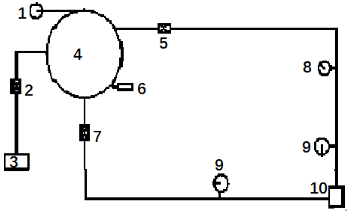1-vacuum gauge; 2-valve switch; 3-vacuum pump; 4-vacuum tank; 5-gate valve; 6-thermometer; 7-gate valve; 8-flow meter; 9-pressure gauge; 10-centrifugal pump

Figure 2. Experimental procedure

In the experiment, the pipeline and the pump were filled with water; the vacuum tank was 75% full of water. The measured water temperature fluctuated between 32℃-34℃, whose effect on the experimental data was slight, so there is no later analysis in this paper. We used the vacuum pump to vacuumize the tank, to control the interworking of the vacuum pipeline and vacuum tank through the valve switch and to determine the value of the air pressure in the vacuum tank by the vacuum gauge reading. When the air pressure in the vacuum tank reached the specified value, the valve was closed. The atmospheric pressure was simulated at different altitudes by controlling the degree of the vacuum, achieving the adjustment of the pump suction inlet pressure, and then realizing the simulation experiment of the flow state in the centrifugal pump in the plateau. The experimental procedure is shown in Figure 2.

An engine drove the centrifugal pump through an accelerator with a growth rate of 1.52. During the experiment, the centrifugal pump shook violently when the engine speed exceeded 1600 r/min. In order not to cause any damage to the equipment, engine speeds of 1100 r/min, 1200 r/min, 1300 r/min, 1400 r/min, 1500 r/min, and 1600 r/min were chosen, so the pump speeds were 1672 r/min, 1824 r/min, 1976 r/min, 2128 r/min, 2280 r/min and 2432 r/min accordingly.

The experiment was carried out in accordance with the methods of Chinese national standards: GB/T 3216-2005 "Rot dynamic pumps-Hydraulic performance acceptance tests-Grade 1 and 2", GB/T18149-2000 "Centrifugal, mixed flow and axial pumps-Code for hydraulic performance tests - Precision class", and GB/T13929-1992 "Methods of testing for water-ring vacuum pumps and compressors".

3. Dara Analysis

The inlet, outlet pressure and flow rate data of the centrifugal pump were obtained by experiments at altitudes of 0m,1000m, 1500m, 2000m, 2500m, 3000m, and with speeds of 1672 r/min, 1824 r/min, 1976 r/min, 2128 r/min, 2280 r/min, and 2432 r/min. Because the data obtained was too much, in this paper two representative groups are listed. In the experiment, the pipeline was connected with a quick connector. The connection method could not tolerate negative pressure, so a gas leak occurred at the junction.  Pressure and the flow data noise were caused by this leak. In this paper, this part of the data is removed as invalid data, in order to ensure the accuracy of the experimental conclusions.

3.1 Analysis of pump pressure

Figure 3 depicts the characteristic of the pump inlet and outlet pressure at different speeds and altitudes, and takes the data of an altitude of 0m and speed of 2280r/min as examples to illustrate the experimental process. The experiment began at 0s while the pump inlet pressure (curve 2) was slightly higher than the pump outlet pressure (curve 1). Then the pump started to work at about 200s where there was a severe pressure fluctuation (circle A, B). Then the pump outlet and inlet pressure tended to be stable until violently fluctuating again at 2300s when the centrifugal pump was not working, the outlet pressure decreased rapidly and the inlet pressure rose (circle C). After a period of time the outlet and inlet pressure reverted to their initial state.

## 3a.png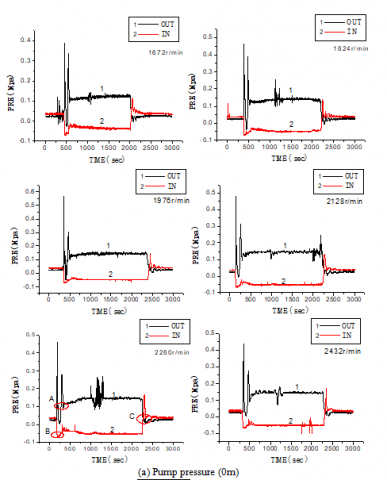## 3b.pngFigure 3. Pump pressure at different altitudes

This paper analyses the inlet pressure Pr and outlet pressure Pc at different speeds and altitudes, calculates the provided pump pressure P, and compares it with the data at an altitude of 0m, so as to obtain the pump pressure decline rate D, and finally, obtains the changing law of the average inlet and outlet pressure. The pressure of the pump and the pump pressure declined at different altitudes and speeds, as shown in Figures 4-7.

## 4.pngFigure 4. Changing rule for the inlet pressure

## 5.png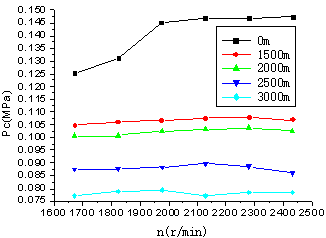Figure 5. Changing rule for the outlet pressure

It can be seen from figure 4 that when the altitude is 0m, the inlet pressure of the pump has a clear downward trend before the speed is over 2000r/min, and remains stable when it drops to about -0.051MPa, while the inlet pressure of the pump at other altitudes does not show this trend. This indicates that the centrifugal pump suction capacity in the low speed zone allows for a certain margin. As the altitude increases, the suction pressure declines due to the decrease of the atmospheric pressure consumed this part of the surplus, which in one way explains the reduction in pump suction capacity. On the other hand, by contrasting the data at different altitudes, we can see that the inlet pressure continued to decrease with the increase in altitude, and the inlet pressure dropped by about 3%-5% with each 500m increase in altitude. The pump inlet pressure decreased correspondingly with the increase in altitude, which indicates that the decrease of suction capacity led to the change in the conditions under which the pump functioned.

Figure 5 shows the changing rule for the outlet pressure, and it can be seen that when the altitude was 0m, the outlet pressure grew rapidly when the speed was lower than 2000r/min and then maintained a steadily increasing trend. This situation is basically consistent with the pump inlet pressure changing rule. This may indicate that the inlet pressure declines or the suction capacity decline has a significant effect on the pump outlet pressure. Compare the outlet pressure data at different altitudes with the same speed, and it can be seen that the outlet pressure decreased quickly with the increase in altitude, and the higher the altitude is, the greater is the decline in the outlet pressure. The outlet pressure drops by about 5%-10% with each 500m increase in altitude.

The pump provided pressure is in accordance with the following changing rules.

## 6.png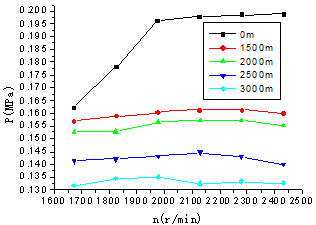Figure 6. Changing rule for provided pressure

At each altitude, the pump provided pressure increases as the speed increases, but the magnitude of the increase is limited when the altitude is over 1500m. When the altitude is over 1500m and the speed is more than 2100r/min, there is even a weak downward trend in the pressure. The rate of decline of the pump provided pressure increases with the speed, and thus the decreasing rate of pump provided pressure in a high-speed zone is higher than that in a low speed zone. Take the data of pump provided pressure at 2000m for example; it decreases by 5.86%, 14.19%, 20.14%, 20.75%, 20.85% and 21.83% for every speed (from low to high) accordingly, and demonstrates a rising tendency.

At the same speed, altitude is inversely proportional to the pump provided pressure. With the elevation in altitude, the pressure that the pump can provide continues to reduce. The provided pressure of the pump of 1824r/min is 0.1589Mpa when the altitude is 1500m, decreasing by about 10.83% compared with 0m. The pump provided pressure drops by 14.19% at 2000m, and the data is 24.69% at 3000m which only reaches 75% of the provided pressure at 0m.

At each altitude, the changing rule of the rate of decline in the pressure tends to be the same, and demonstrates a trend of rapid increase and then flattening out. But we can see directly that the higher the altitude is, the greater the pump pressure rate of decline is. The pump pressure rate of decline is significantly higher in a high-speed zone than in a low speed zone. The rate of decline in the pressure is about 2%-6% with each 500m increase in altitude.

## 7.pngFigure 7. Changing rule for the rate of decline in the pressure

3.2 Analysis of pump flow rate

Figure 8 describes the pump flow rate data under different working conditions. Taking the data of 0m and 2280r/min for example, the centrifugal pump starts to work at 500s, and the pump flow rate increases rapidly (circle A) and then remains at a relatively stable state until the pump ceases to work at 2500s, then the flow rate drops quickly (circle B), at last it gradually returns to the initial stage.

## 8a.png## 8b.png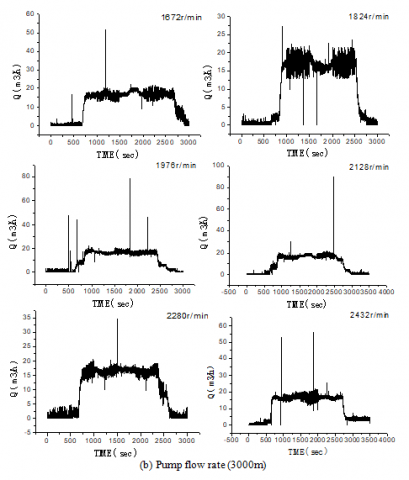Figure 8. Pump flow rate data at different altitudes

The maximum and the minimum flow rate under different steady conditions are acquired according to the flow rate curves. Taking the average value of the pump flow rate Q at different altitudes and speeds and then comparing it with the flow rate data at 0m, we can discover the average flow rate decline rate u under different working conditions and calculate the changing rule, as shown in Figures 9-10.

## 9.png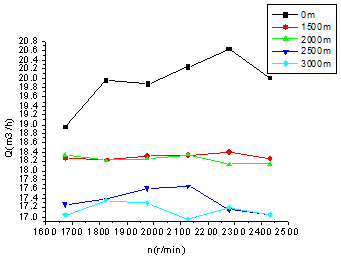Figure 9. The changing rule of the flow rate

The variation law can be discovered from analyzing the flow rate data in figure 9.

The higher the altitude is, the lower the flow rate is at each speed. The flow rate of the pump shows a downward trend with the elevation in altitude. Taking data of 1976r/min for example, the pump flow rate is 19.877m3/h when the altitude is 0m, while the flow rate of 1500m is 18.313m3/h, which is about 7.87% lower. At an altitude of 2000m and 3000m, the pump flow rate decreases by 8.19% and 13.03%. Thus, with the elevation in altitude, the flow rate of the pump is continuously reduced.

With the increase in altitude, the faster the speed is, the more greatly and rapidly the pump flow rate decreases. We can discover this by analyzing the data of 2500m, where the average flow rate at all kinds of speed is 17.270m3/h, 17.375m3/h, 17.604m3/h, 17.658m3/h, 17.145m3/h, 17.037m3/h, decreasing by 8.83%, 12.96%, 11.44%, 12.81%, 16.90%, 14.88% respectively compared with the flow at 0m. When compared with the data at other altitudes, these rules also apply. Thus, the rate of decline of the pump flow in a high speed zone is significantly greater than in a low speed zone.

## 10.png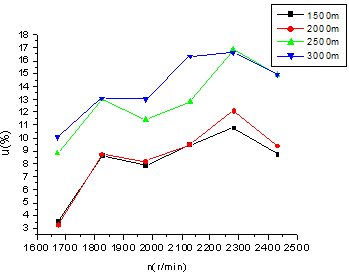Figure10. Changing rule of the rate of decline in the flow

Through the analysis of the changing rule for the rate of decline in the flow, it can be seen that the rate increases with the increase in speed and altitude, but does not show a law of linear growth. There is even a partial fall phenomenon when the speed is between 1800r/min-2000r/min and 2300r/min-2500r/min, while the pump flow rate decreases most rapidly when the revolution is between 2128r/min and 2280r/min. The causes of this phenomenon require follow-up experiments to explore further, and there is no in-depth study of this phenomenon in this paper.

3.3 Analysis of pump efficiency

Taking the pump efficiency data at 0m as the reference datum and supposing it to be 100, the change in the rate of pump efficiency can be calculated at different altitudes and then the changing rule of decline in the pump efficiency E under each work condition can be obtained, as shown in Figure 11.

## 11.png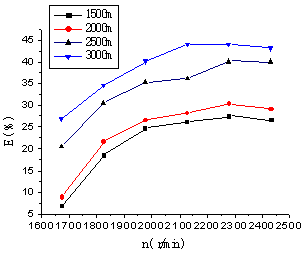Figure 11. Changing rule of decline in pump efficiency

Seen in figure 11, at the same altitude, the rate of the decline in pump efficiency in a high-speed zone is bigger than that in a low speed zone; at the same speed, the higher the altitude is, the lower the efficiency of the pump is and the more its efficiency decreases. At an altitude of 3000m and a speed of 2128-2280r/min, the pump efficiency drops by about 44%. This also means that with the change in centrifugal pump hydraulic characteristics, the pump's high efficiency zone has been significantly offset and becomes equivalent to a low speed zone. The pump efficiency is reduced by about 2%-9% with every 500m increase of altitude in this research.

3.4 Analysis of reasons for the decline in pump hydraulic characteristics

Based on the theory of fluid mechanics, a simple mathematical model of the pump is established to analyze the reasons from the perspective of energy conservation. The inlet and outlet of the pump satisfy the energy conservation law:

$C=0.5 \rho v_{\mathrm{in}}^{2}+P_{in}+\mathrm{W}=0.5 \rho v_{o u t}^{2}+P_{o u t}+Z+S$    (1)

Where: ρ is fluid density; vin is inlet velocity; Pin is inlet pressure; W is pump shaft power; vout is outlet velocity; Pout is outlet pressure; Z is pump inlet and outlet height difference; S is pump energy consumption.

First, we assume that the pump speed is constant, and then the pump inlet velocity vin and shaft power W can be considered to be basically unchanged. At the same time, when the altitude is elevated, that means the inlet pressure Pin is decreased, so the total energy C decreases. Checking the right side of the equation, for a particular pump, the inlet and outlet height difference Z is constant, and research in reference  shows that the energy consumption of gas burnt S increases with the elevation. In order to keep the balance of the equation, the pump outlet pressure Pout needs to be reduced, which appears as the reduction of pump head; or the kinetic energy of the pump outlet fluid 0.5vout2 needs to be reduced, which appears as a decline in the flow rate.

The inlet liquid content of the pump declines in a plateau as shown in reference . According to the analysis of the liquid content, it can be seen that it decreases along with the increase in speed. Due to the high compressibility of the gas, the air bubbles gather some energy in the blade compression process. After entering the pump shell, the pressure inside the pump shell is reduced, the expansion and burst of bubbles consume this part of the energy, and the pressure of the liquid is consequently reduced, which shows as a decline in the pump outlet pressure. Because the higher the speed is, the more the air bubbles are compressed, and the more the concentration and consumption energy costs, the more clearly the pump head decreases. These reasons can be summarized as an increase in frictional losses within the pump, which leads to the reduction of the pump hydraulic characteristics.

The pump efficiency is the ratio of the effective power to the shaft power, and the formula is as follows:

$\eta=\frac{\rho g q h}{P}$     (2)

where: ρ is density, (kg/m3); g is gravitational acceleration, (m/s); q is pump flow, (m3/s); h is pump head, (m); and P is shaft power, (kW).

It can be seen from the analysis above that the flow rate and the pressure of the centrifugal pump are decreased with the increase in altitude, yet the engine speed and the pump shaft power stay constant, which will inevitably lead to a reduction in pump efficiency.

Besides, it can be observed from the pump pressure and flow rate curves that when the altitude is less than 2000m, the curve is relatively smooth; but it fluctuates increasingly fiercely with the increase in the vacuum. This also shows that the pump fluid is not smooth and the working state is no more stable. The violent vibration of the fluid increases the friction resistance and energy loss, and finally leads to a decline in the pump efficiency.

4. Conclusion

In this paper, experiments of the hydraulic characteristics of a centrifugal pump in a plateau are carried out through the method of controlling the inlet vacuum of the centrifugal pump, and data including the inlet and outlet pressure and the flow rate were collected. By analyzing these data, the following conclusions are reached:

The higher the altitude is, the bigger the pump inlet, outlet and provided pressure drops. The decline rate in a high speed zone is higher than that in a low speed zone. The pump pressure drops by about 5%-10% with every 500m increase in altitude. The inlet pressure decline has a significant effect on the pump hydraulic characteristics.

As the altitude increases, the pump flow rate decreases. The rate of decline in the pump flow basically produces a linear increase with the same speed. At the same altitude, the flow rate decline in a high speed zone is generally higher than that in a low speed zone. The pump flow rate decreases by about 2%-5% with every 500m elevation of altitude.

Altitude has a great influence on the pump efficiency. The efficiency of the pump decreases by about 2%-9% with every 500m elevation of altitude. The pump efficiency decreases most quickly when revolutions range from 2128r/min to 2280r/min. The pump's high efficiency zone has been significantly offset and tends to be equivalent to a low speed zone in a plateau.

The experimental results indicate that the hydraulic characteristics of the pump are significantly changed in a plateau, especially at high altitude and a high-speed zone. The theoretical analysis results demonstrate that the increase of pump frictional loss is the fundamental reason for the hydraulic characteristics change of the centrifugal pump in a plateau. The centrifugal pump needs to be adjusted accordingly in order to adapt to the plateau environment.

The results of this paper are an effective complement to the theory of fluid transportation in a plateau, and have a guiding significance and theoretical value for the improvement of the existing centrifugal pump and the development of a new type of centrifugal pump.

Acknowledgment

This research is supported by the Chongqing Postdoctoral Fund (No. Xm2016026).

References

 Li J., Zhang W.M., Li T. (2013). Research on engine-pump units adaptability in the plateau, Advanced Materials Research, Vol. 614-615, pp. 371-375. DOI: 10.4028/www.scientific.net/AMR.614-615.371

 Shankar V.K.A., Umashankar S., Paramasivam S., Hanigovszki N. (2016). A comprehensive review on energy efficiency enhancement initiatives in centrifugal pumping system, Applied Energy, No. 181, pp. 495-513. DOI: 10.1016/j.apenergy.2016.08.070

 Barbarelli S., Amelio M., Florio G. (2016). Predictive model estimating the performances of centrifugal pumps used as turbines, Energy, No. 107, pp. 103-121. DOI: 10.1016/j.energy.2016.03.122

 Kaliakatsos D., Cucumo M., Ferraro V., Mele M., Galloro A., Accorinti F. (2016). CFD analysis of a pipe equipped with twisted tape, International Journal of Heat and Technology, Vol. 34, No. 2, pp. 172-180. DOI: 10.18280/ijht.340203

 Li J., Zhang W.M., Yang X.J., Wu J.J. (2013). Experimental research on performance of aircraft tanker truck with different oil pump in the plateau, Applied Mechanic & Meterials, Vol. 405-408, No. 6, 3231-3234. DOI: 10.4028/www.scientific.net/AMM.405-408.3231

 Schäfer T., Bieberle A., Neumann M., Hampel U. (2015). Application of gamma-ray computed tomography for the analysis of gas holdup distributions in centrifugal pumps, Flow Measurement & Instrumentation, No. 46, pp. 262-267. DOI: 10.1016/j.flowmeasinst.2015.06.001

 Shi B.C., Wei J., Zhang Y. (2017). A novel experimental facility for measuring internal flow of Solid-liquid two-phase flow in a centrifugal pump by PIV, International Journal of Multiphase Flow, No. 89, pp. 266-276. DOI: 10.1016/j.ijmultiphaseflow.2016.11.002

 LI Y.L., Yuan S.Q., Tang Y., Yuan J.P. (2012). Motion of tracer particles in a centrifugal pump and its tracking characteristics, Journal of Hydrodynamics, Vol. 24, No. 5, pp. 785-793. DOI: 10.1016/S1001-6058(11)60304-1

 Bounaouara H., Ettouati H., Ticha H.B., Mhimid A., Sautet J.C. (2015). Numerical simulation of gas-particles two phase flow in pipe of complex geometry: pneumatic conveying of olive cake particles toward a dust burner, International Journal of Heat and Technology, Vol. 33, No. 1, pp. 91-98. DOI: 10.18280/ijht.330114

 Caridad J., Asuaje M., Kenyery F., Tremante A., Aguillón O. (2008). Characterization of a centrifugal pump impeller under two-phase flow conditions, Journal of Petroleum Science & Engineering, Vol. 63, No. 1, pp. 18-22. DOI: 10.1016/j.petrol.2008.06.005

 Pei J., Yuan S.Q, Li X.J., Yuan J.P. (2014). Numerical prediction of 3-D periodic flow unsteadiness in a centrifugal pump under part-load condition, Journal of Hydrodynamics, Vol. 26, No. 2, pp. 257-263. DOI: 10.1016/S1001-6058(14)60029-9

 Liu H.L., Liu D.X., Wang Y., Wu X., Wang J. (2013). Application of modified k-ω model to predicting cavitating flow in centrifugal pump, Water Science and Engineering, Vol. 6, No. 3, pp. 331-339. DOI: 10.3882/j.issn.1674-2370.2013.03.009

 Kalteh M., Abbassi A., Saffar-Avval M., Harting J. (2011). Eulerian two-phase numerical simulation of nanofluid laminar forced convection in a microchannel, International Journal of Heat & Fluid Flow, Vol. 32, No. 1, pp. 107-116. DOI: 10.1016/j.ijheatfluidflow.2010.08.001

 Rafiee S.E., Sadeghiazad M.M. (2016). Three-dimensional CFD simulation of fluid flow inside a vortex tube on basis of an experimental model- the optimization of vortex chamber radius, International Journal of Heat and Technology, Vol. 34, No. 2, pp. 236-244. DOI: 10.18280/ijht.340212

 Li J., Zhang W.M. (2014). Effect of altitude on suction performance of centrifugal pump, Journal of Logistical Engineering University, Vol. 30, No. 5, pp. 36-40.